# How to Find a Pair of Element with Sum K in a Sorted Array

## How to Find a Pair of Elements with Sum K in a Sorted Array:

In this article, I am going to discuss How to Find a Pair of Elements with Sum K from a sorted Array in C Language with Examples. In our previous article, where we discussed Finding a Pair of Element with Sum K from an Unsorted Array in C Language with Examples.

##### Finding a Pair of Element with Sum K in a Sorted Array:

We have to find a pair of elements in a sorted array such that their total will equal to some given number. Let’s take an example:

A + B = 10

We have to find A and B so that their sum will equal to 10.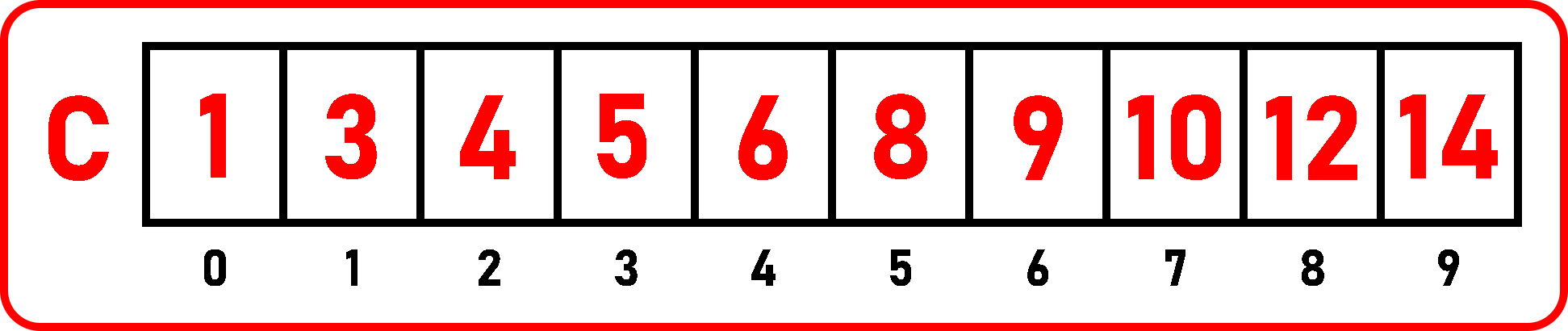Here, we have taken a sorted array of size 10. So, first, we have to check whether there are any elements or not. As we can see, there are some elements whose pair will give the sum of 10, i.e. 1 and 9, 6 and 4.

We have found two pairs of elements. As the array is already sorted, smaller elements are present on the left side, and greater elements are present on the right side. So, whenever we found a pair, then one number will be smaller and one number will be greater i.e. 1 and 9, 6 and 4. So, we can start scanning the array from both directions to find one small element and one large element.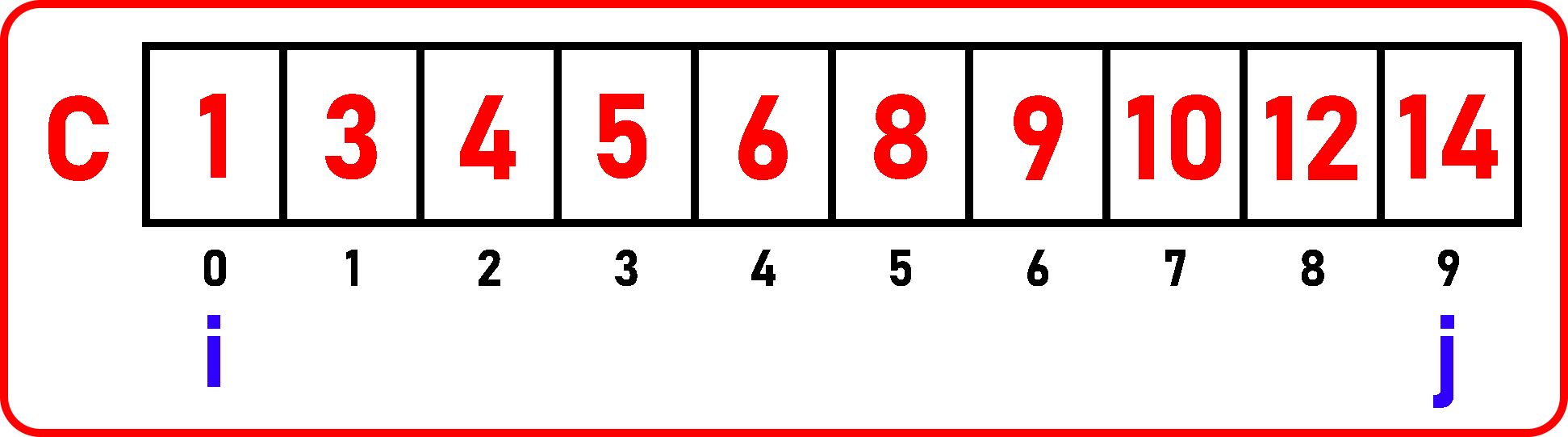We will initialize i variable to the 0th index of the array and the j variable to the last index of the array. i will point to small elements and j will point to greater elements.

Now for scanning the array with both directions, we will increment i and decrement j. And every iteration we will check whether C [i] + C [j] = 10? If yes print those elements. If we got the sum of C [i] + C [j] = 10, then we decrement j because the sum is greater so we have to shift a smaller number on the right side.

Here, C [i] + C [j] = 10 is 1 + 14 = 15, which is > 10. So, decrement j.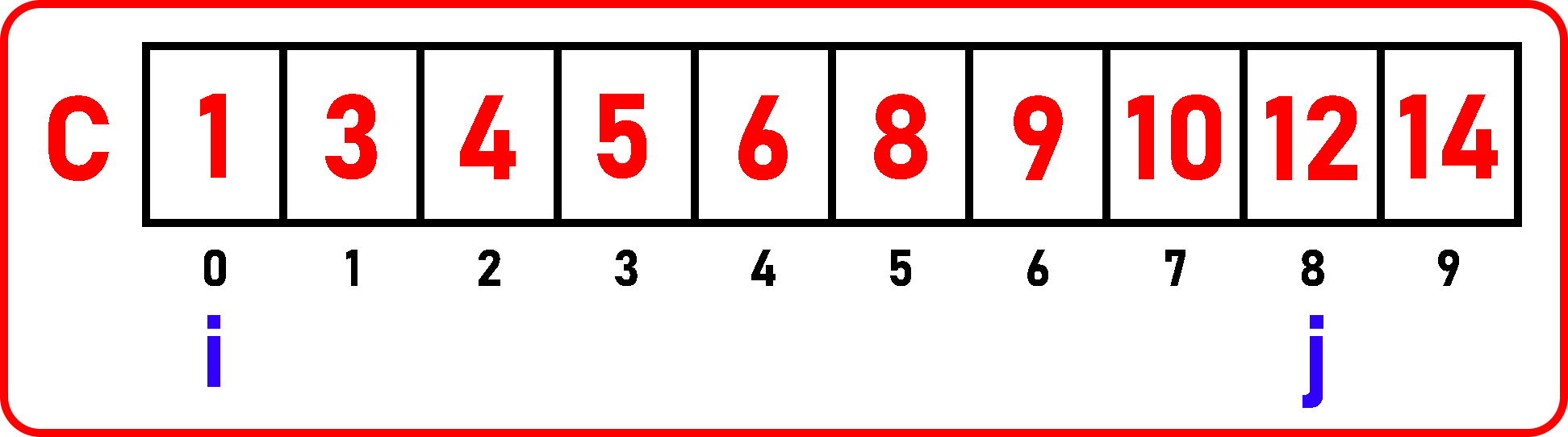Now, C [i] + C [j] = 10 is 1 + 12 = 13, again > 10. Decrement j.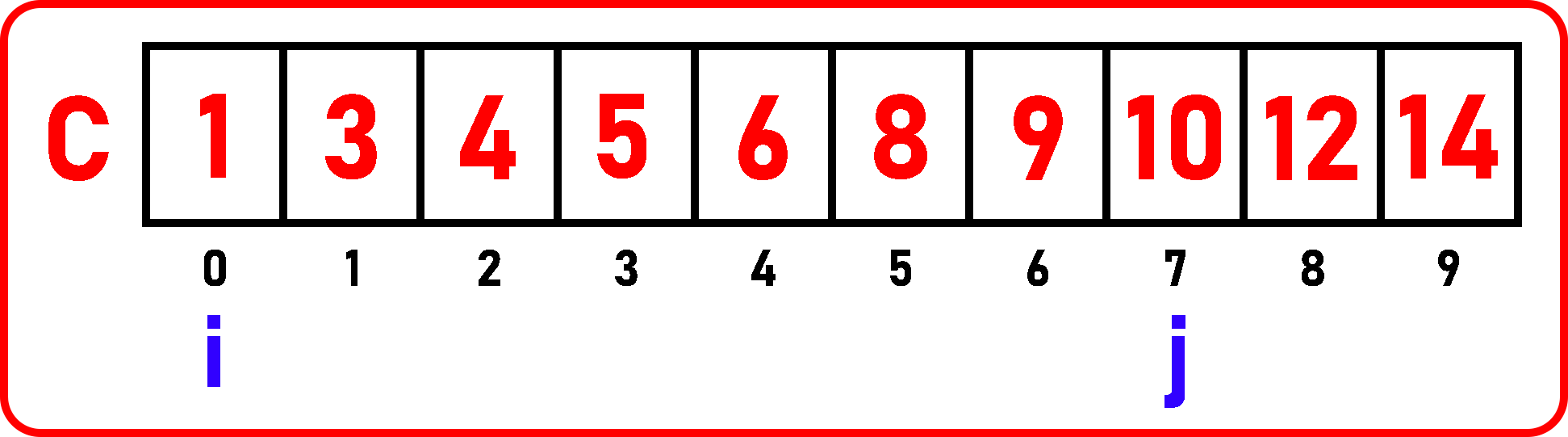Here, C [i] + C [j] = 10 is 1 + 10 = 11, again > 10. Decrement j.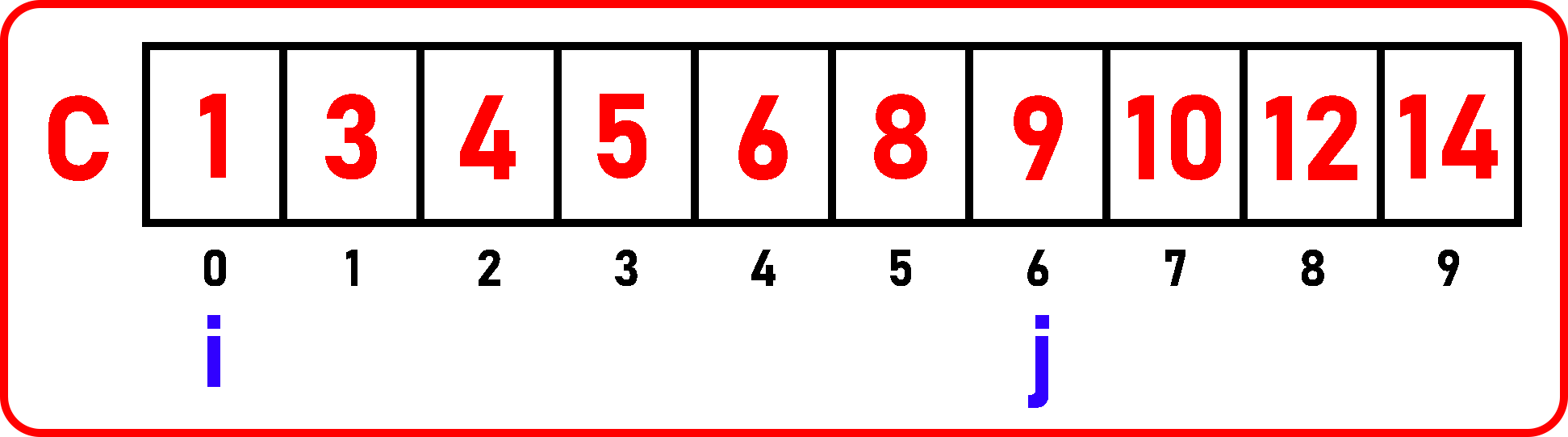Here, C [i] + C [j] = 10 is 1 + 9 = 10. Here we found a match so print these elements and decrement j and increment i.

Note: when we found a match decrement j as well as increment i.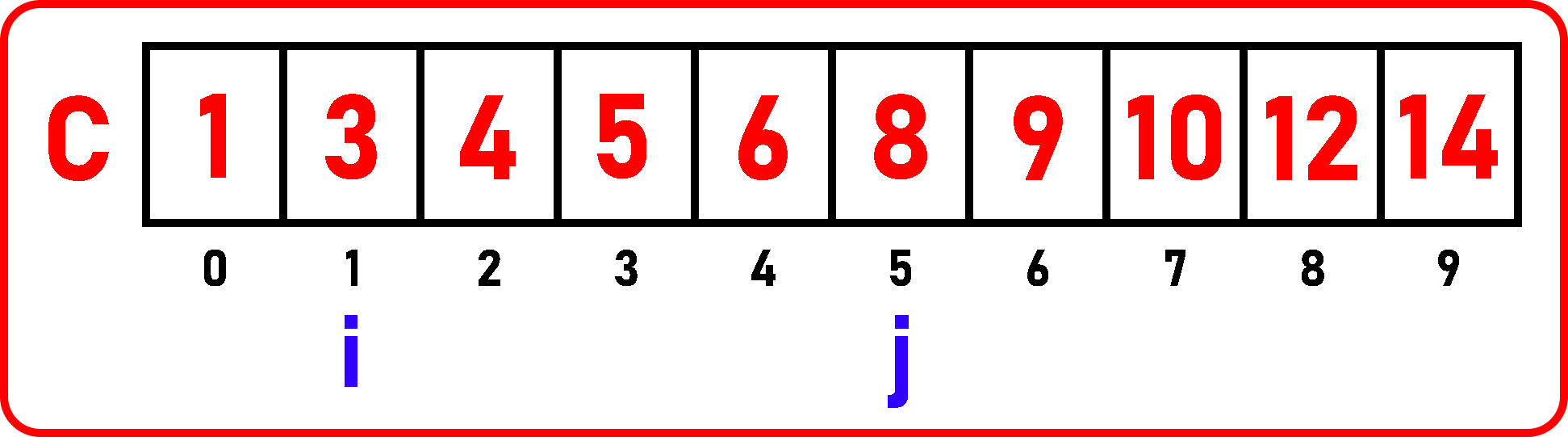Check again if C [i] + C [j] = 10, 3 + 8 = 11, its > 10. Decrement j.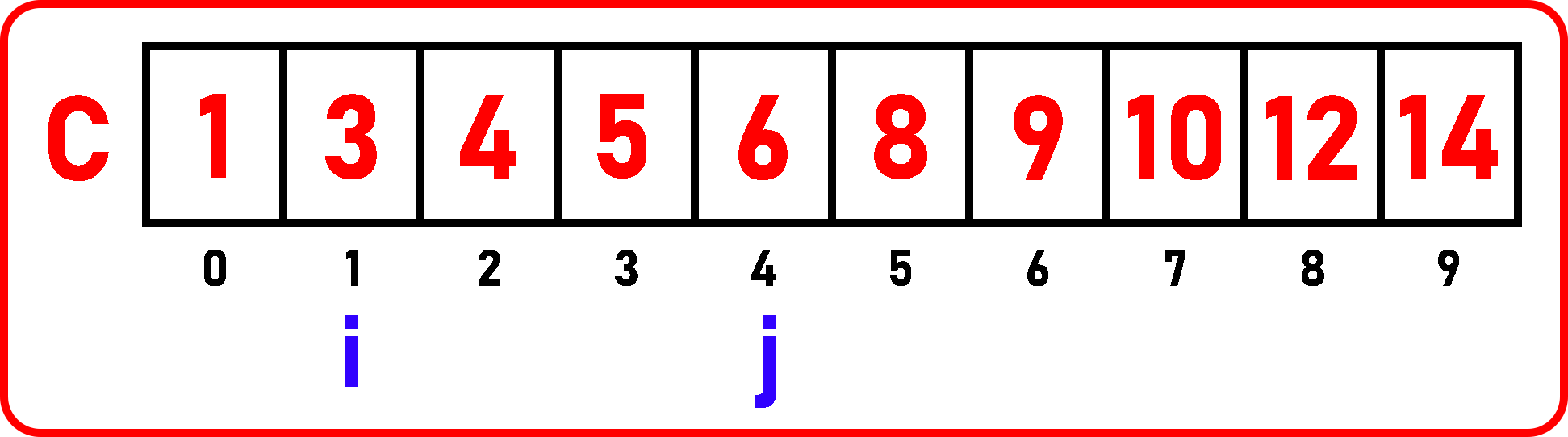Check if C [i] + C [j] = 10,

3 + 6 = 9, it is < 10. Here the sum is less than 10 so don’t decrement j. Now, we have to increment i only.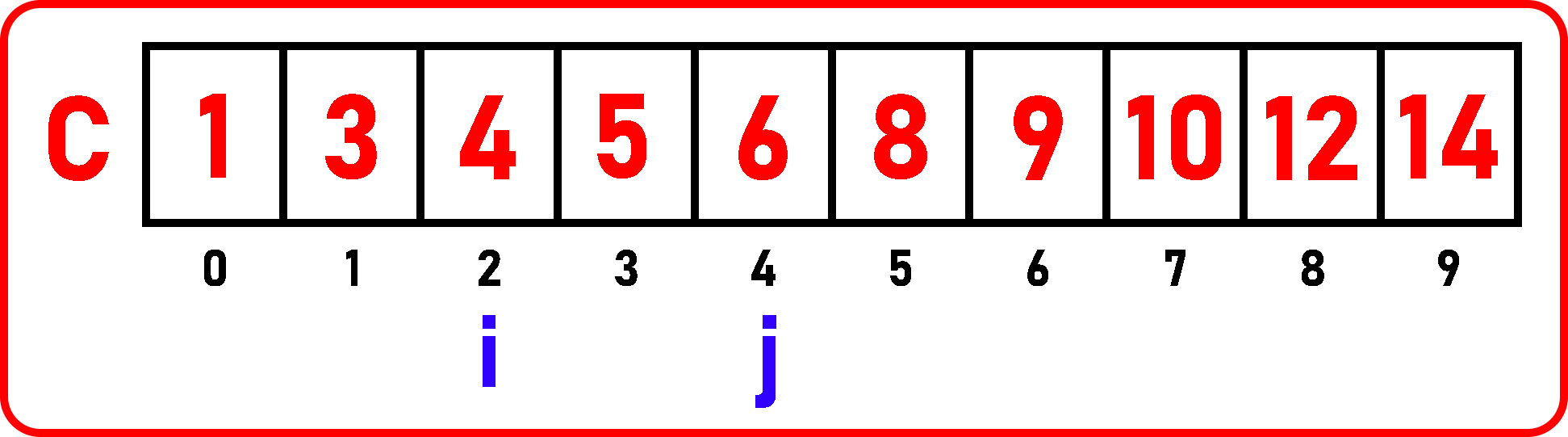Now, check if C [i] + C [j] = 10,

4 + 6 = 10. Here we found a match so print these elements and decrement j and increment i.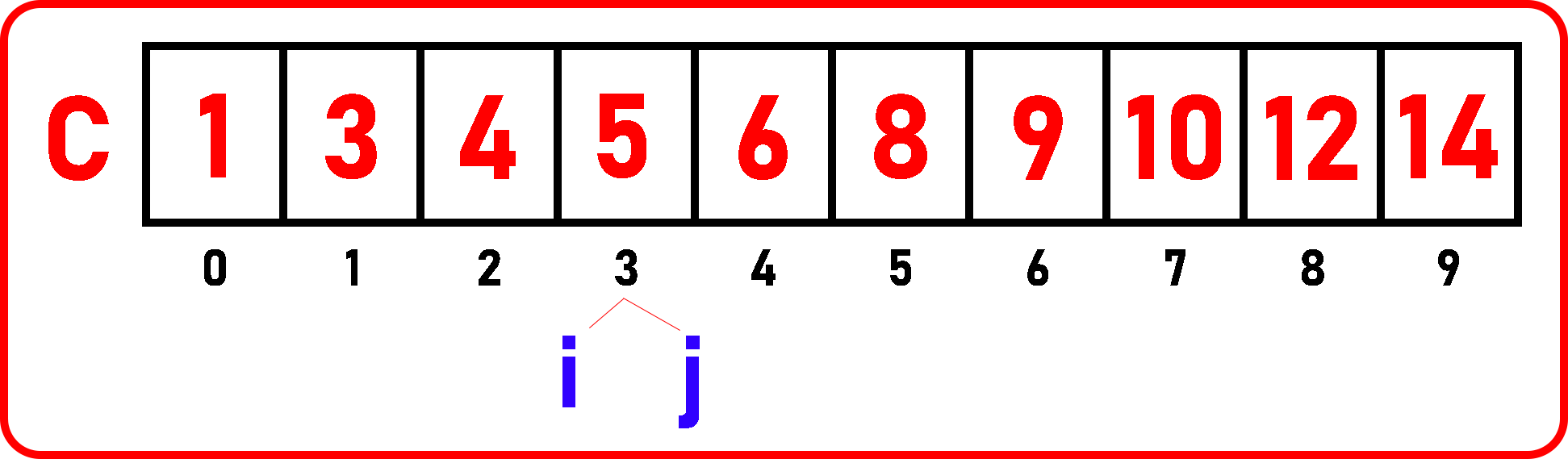Now, i and j pointing on single element, so pairing is not possible. Here we terminate our procedure. In simple words, what we did:

If C [i] + C [j] > GIVEN_NUM, then decrement j.

If C [i] + C [j] < GIVEN_NUM, then increment i.

If C [i] + C [j] = GIVEN_NUM, then decrement j and increment i.

Note: we will use a while loop here because we are not incrementing or decrementing i and j every time. We have to increment or decrement only if any of the given conditions will match.

##### Finding a Pair of Element with Sum K in a Sorted Array Code in C language:

USING WHILE LOOP-

```#include <stdio.h>
#include <stdlib.h>
struct List{
int C;
int size;
int length;
};

void Display(struct List list) {
int i;
printf("Elements are:\n");
for (i = 0;i<list.length;i++)
printf("%d ", list.C[i]);
printf("\n\n");
}

void SumPairSorted(struct List list, int sum){
int i = 0, j = list.length - 1;

while (i < j){
if(list.C[i] + list.C[j] == sum){
printf("%d + %d = %d\n", list.C[i], list.C[j], sum);
i++;
j--;
}
else if(list.C[i] + list.C[j] < sum){
i++;
}
else{
j--;
}
}
}

int main(){
struct List list_1 = {{1, 3, 4, 5, 6, 8, 9, 10, 12, 14}, 10, 10};
Display(list_1);
SumPairSorted(list_1, 10);
}
```
###### USING FOR LOOP-
```#include <stdio.h>
#include <stdlib.h>

struct List{
int C;
int size;
int length;
};

void Display(struct List list) {
int i;
printf("Elements are:\n");
for (i = 0;i<list.length;i++)
printf("%d ", list.C[i]);
printf("\n\n");
}

void SumPairSorted(struct List list, int sum){
int i, j;

for(i = 0, j = list.length - 1; i < j;){
if(list.C[i] + list.C[j] == sum){
printf("%d + %d = %d\n", list.C[i], list.C[j], sum);
i++;
j--;
}
else if(list.C[i] + list.C[j] < sum){
i++;
}
else{
j--;
}
}
}

int main(){
struct List list_1 = {{1, 3, 4, 5, 6, 8, 9, 10, 12, 14}, 10, 10};
Display(list_1);
SumPairSorted(list_1, 10);
}

```

Time Complexity: O (n)

###### Output: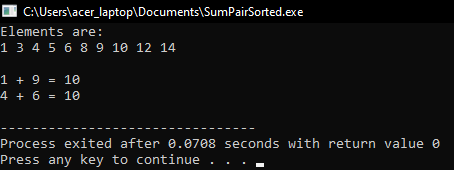In the next article, I am going to discuss How to Find Maximum and Minimum Elements present in an array in C Language with Examples. Here, in this article, I try to explain How to Find a Pair of Elements with Sum K in a Sorted Array using C Language with Examples and I hope you enjoy this Finding a Pair of Elements with Sum K in a Sorted Array using C Language with Examples article.